# Fourier Transform Spectroscopy

## Introduction

A Fourier transform spectrometer (FTS) is an optical device that utilizes an interferometer with a translating mirror to produce an interferogram. A Fourier transform of the interferogram provides the spectrum of the source. FTS methods are often preferred over monochromators due to increased measurement speed, increased resolution, and mechanical simplicity . Modeling a FTS in FRED is not complicated. In this example, a FTS model will be built and operated in FRED using an Embedded Script. Three different spectra will be analyzed using this model.

## Building the Spectrometer in FRED

To simplify the process, an ideal point source, ideal lens, and ideal beamsplitting surface are used (Figure 1). Detailed extended sources, real lenses, beamsplitting cubes, or wiregrid beamsplitters can be incorporated for more realistic analysis.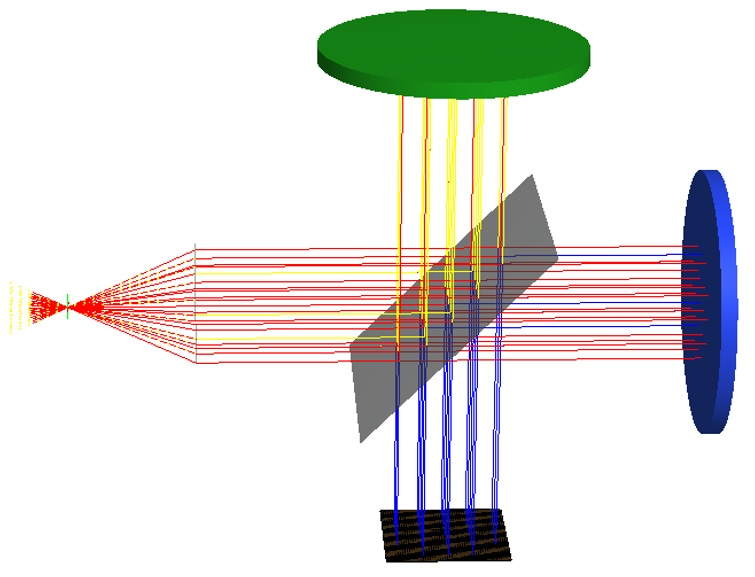Figure 1Simple Fourier transform spectrometer model, consisting of a point source, ideal lens, and Michelson interferometer with a moving mirror. Collimated light from the source is sent into a 50/50 beamsplitter. Reflected light is directed towards a fixed mirror (green), and transmitted light is directed towards a translating mirror (blue). Light from both paths are recombined after the beamsplitter, and collected power is measured at the detector (black).

The first step in the FRED model is to create a coherent point source object. Next, a spectrum is created and assigned to the source. The spectrum can be imported from a text document, digitized from a graph image, or calculated from a specific function (Gaussian or blackbody). The ideal lens is created using FRED’s “Custom Element” object consisting of a “Lens Module” surface type located a distance of 10 mm from the point source. The “Lens Module” surface has focal length of 10 mm and semi aperture of 5 mm. Next, the ideal beamsplitting surface is created using a plane surface rotated at 45 degrees from the collimated beam. A custom “50/50” beamsplitting coating (Figure 2) is created and applied it to this surface.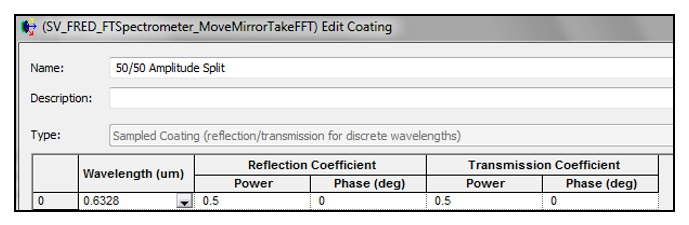Figure 2Custom 50/50 beamsplitter coating specifications. If a single wavelength is specified, the coating will apply identically to all wavelengths in the source.

The two mirrors in the system are created by using two of FRED’s “Mirror” objects both with the “Reflect” coating and “Reflect All” Raytrace Control. Each mirror is located a distance of 20 mm from the beamsplitter, one translated in the +y-direction and the other in the +z-direction. Finally, the model is completed with the addition of an absorbing surface and corresponding analysis surface normal to the combined beam exiting the beamsplitter.

Operating Spectrometer to Recover Source Spectrum

In this initial configuration, the two beam paths in the interferometer have identical path lengths for an optical path difference (OPD) of zero. To gather spectral information of the source, one mirror must translate some distance to change OPD. At each step of the mirror’s motion, power on the detector will be collected. The resulting graph of detector power vs. OPD, called an interferogram, will undergo a fast Fourier transform (FFT) to determine source power vs. light spatial frequency. To automate this process, an Embedded Script (written in FRED’s built-in BASIC) can be created to move the mirror and collect detector values.

In the following examples, the translating mirror is moved a total distance of 0.04 mm with 1024 steps. For the sake of the FFT algorithm, the number of steps must be a power of two. The higher the resolution, the more accurate the resulting spectrum. Low resolution mirror scans run the risk of undersampling the interferogram. An undersampled interferogram can lead to aliasing and artificially low spatial frequencies in the FFT. Light spectra are assigned to the source as “evenly spaced, weighted according to spectrum”. It is also important to sample the spectrum sufficiently. In this example, the maximum number of wavelength samples, 256, is used. Undersampled source spectra lead to cosine fringes in the reproduced spectrum.

Example 1: Single-Wavelength Source

The source is given a single wavelength of 1.5 µm. This wavelength corresponds to a spatial frequency of 666.7 (1/mm). The interferogram of a single wavelength is simply a cosine function. (Figure 3).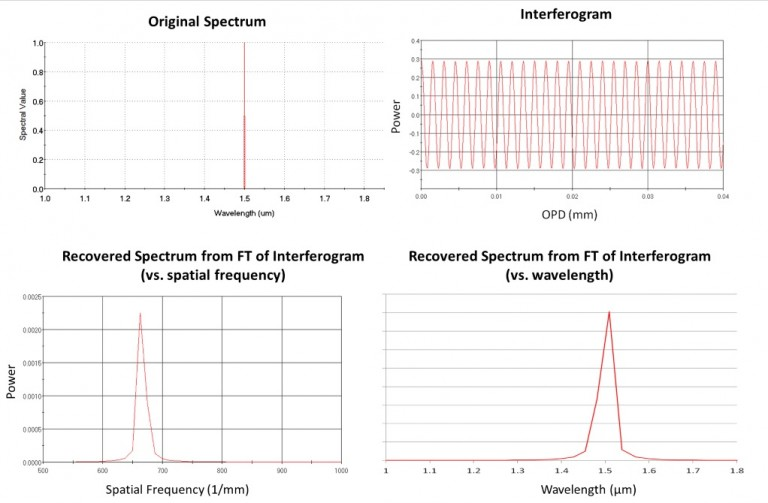Figure 3Single-wavelength source spectrum evaluated by a FTS. Top left: original source spectrum plotted vs. wavelength in µm. Top right: Interferogram showing detector power vs. OPD between light paths of interferometer. Bottom left: Recovered source spectrum plotted vs. spatial frequency in 1/mm. Bottom left: Recovered source spectrum plotted vs. wavelength in µm.

Example 2: Gaussian Spectrum

The source is given a Gaussian spectrum with center wavelength of 0.555 µm and half width at half max of 0.0589 µm. The central wavelength corresponds to a spatial frequency of 1801.8 (1/mm). Note that the recovered spectrum has an apparent asymmetry (Figure 4). This is because the spectrum is a Gaussian function of wavelength, and spatial frequency the inverse of wavelength. Shorter spatial frequency intervals correspond to a larger range of wavelengths; thus, the left half of the recovered spectrum will appear more compressed. (Figure 4).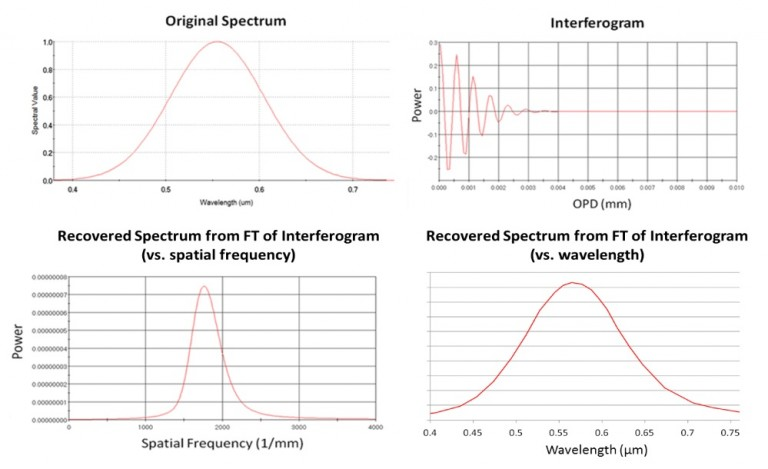Figure 4Gaussian source spectrum evaluated by a FTS. Top left: original source spectrum plotted vs. wavelength in µm. Top right: Interferogram showing detector power vs. OPD between light paths of interferometer. Bottom left: Recovered source spectrum plotted vs. spatial frequency in 1/mm. Bottom left: Recovered source spectrum plotted vs. wavelength in µm.

Example 3: White LED Spectrum

The source is given a spectrum characteristic of a white light phosphor LED. The spectrum has a sharp peak at wavelength=0.45 um and a broad peak at wavelength=0.65 um. The blue peak corresponds to a spatial frequency of 2222.2 (1/mm). The red peak corresponds to a spatial frequency of 1538.5 (1/mm). Note that the recovered spectrum shape is flipped horizontally (Figure 5). This is because the spectrum is a function of wavelength, and spatial frequency the inverse of wavelength. Additionally, the recovered spectrum shows higher low-spatial frequency values compared to the red peak of the original spectrum. This is because low spatial frequency intervals correspond to a larger range of wavelengths, and more light power will be integrated over these spatial frequencies.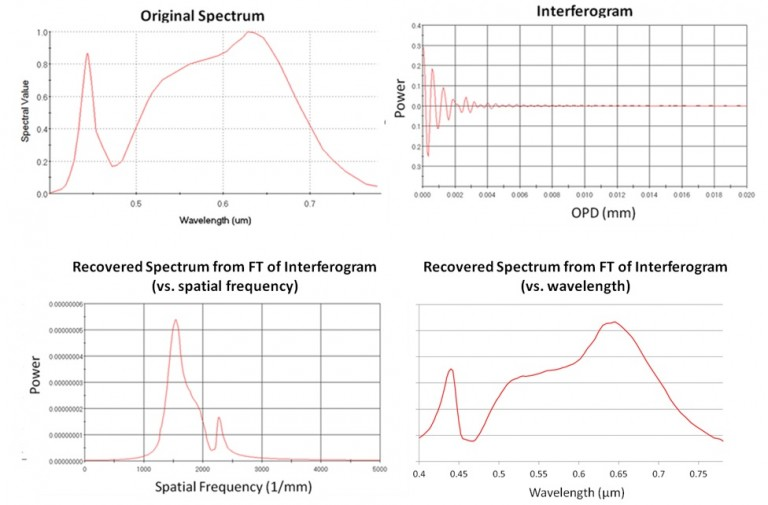Figure 5White LED source spectrum evaluated by a FTS. Top left: original source spectrum plotted vs. wavelength in µm. Top right: Interferogram showing detector power vs. OPD between light paths of interferometer. Bottom left: Recovered source spectrum plotted vs. spatial frequency in 1/mm. Bottom left: Recovered source spectrum plotted vs. wavelength in µm.

 “Introduction to Fourier Transform Infrared Spectrometry.” Thermo Nicolet Corporation. 2001. Accessed December 8, 2015.  http://mmrc.caltech.edu/FTIR/Literature/FTIRintro.pdf